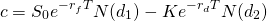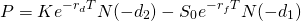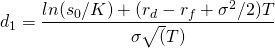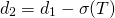# Garman-Kohlhagen model

The Garman-Kohlhagen option pricing model is an option valuation model that can be used to value European currency options. The Garman-Kohlhagen model treats foreign currencies as if they are equity securities that provide a known dividend yield. The owner of the foreign (domestic) currency receives a dividend yield equal to the risk-free rate in the foreign (domestic) country.

Similarly to the Black-Scholes option pricing model, the exchange rate is assumed to follow a Brownian motion. At the bottom of this page, we implement an Excel spreadsheet that implements a Garman Kohlhagen calculator.

## Garman-Kohlhagen model explanation

The Garman-Kohlhagen model is a modification to the Black-Scholes option pricing model. It was proposed in 1983 by Garman and Kohlhagen. Garman and Kohlhagen modified the Black–Scholes model such that the model can cope with the presence of two risk-free interest rates. The difference with the BS model is that GK model corrects the difference between native and foreign interest rates. At the same time, the GK model shares the same limitations as the Black-Scholes model.

The Garman-Kohlhagen model predicts that FX call options are cheaper than standard European call options but FX put options are more expensive than standard put options.

## Garman Kohlhagen model formula

Suppose rd is the risk free rate of the domestic currency and rf is the foreign currency risk free rate. Note that we have to use the FX rate such that both the strike and current spot are expressed in terms of units of domestic currency per unit of foreign currency. The resulting option value we get will also be in the same units.

The domestic currency value of a call option is thenThe put option formula iswhereS0 is the current spot price

K is the strike price

N(x) is the cumulative normal distribution function

rd is the domestic risk free rate

rf is the foreign risk free rate

T is the time to maturity

And sigma is the volatility of the FX rate.

Garman-Kohlhagen example

The Garman-Kohlhagen calculator using an Excel spreadsheet can be downloaded below. In the spreadsheet, we implement an example that illustrates how to use the above formulae.

### Garman-Kohlhagen calculator

Want to have an implementation in Excel? Download the Excel file: Garman-Kohlhagen model